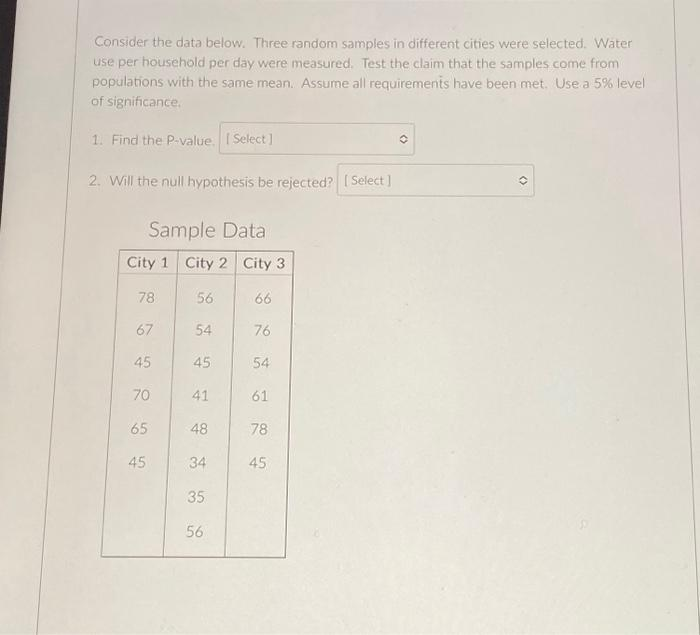Home / Expert Answers / Statistics and Probability / need-help-cant-figure-it-out-consider-the-data-below-three-random-samples-in-different-cities-were-pa219

# (Solved): need help. cant figure it out Consider the data below. Three random samples in different cities were ...

need help. cant figure it outConsider the data below. Three random samples in different cities were selected. Water use per household per day were measured. Test the claim that the samples come from populations with the same mean. Assume all requirements have been met. Use a level of significance. 1. Find the P-value. 2. Will the null hypothesis be rejected? Sample Data

We have an Answer from Expert

Our hypotheses for testing -

H0: The population means across the three cities are equal .

Ha: At least two population means among the three are not equal .

Here number of treatments (k)=3

Total number of observations across three samples (N)=20

For the given testing scenario we will be using F-test with df=(k-1,N-k)=(2,17)

Information on sample from City 1 -
Number of observations (n1)=6
Sum of observations (  370
Sum of square of observations (  =23748

Information on sample from City 2-
n2=8 ,   =369 ,   =17579

Information on sample from City 3-
n3=6 ,   =380 ,   =24878
We have an Answer from Expert